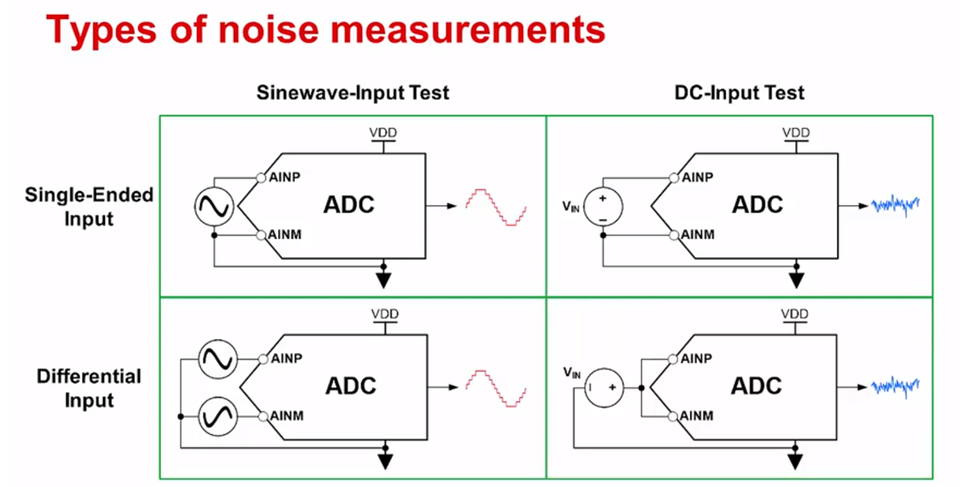If you have a related question, please click the "Ask a related question" button in the top right corner. The newly created question will be automatically linked to this question.

Hi,

I have used ADS131M08 for AC Line Voltage sensing application.

Regards,

Deepak

• Hello Deepak,

Edit: I got my wires crossed what the question was asking, so you probably saw a much different answer in the response email. I've fixed it below.

There's a great video on the subject which can be found here: https://training.ti.com/adc-noise-measurement-methods-parametersAssuming you're using the ADS131M08 GUI, just capture a waveform, navigate to the Spectral Analysis page, and look for the spectrum and other common noise specifications like SNR. If you're not using the EVM, I'd use the [GUI] Analog Engineer’s Calculator  which can take ADC data and calculate the same values. This is found on the Data Analysis Tool Kit (FFT/Hys) page. If you have some other MATLAB signal analysis tools, you can use that too.

A small note that DC performance and AC performance might be a bit different so if you have a frequency of interest, I suggest taking data at that frequency of interest. For example, this device is very popular for eMetering applications which utilize 50/60Hz. So a good way to test for that noise is to apply a 50 or 60 Hz signal with an amplitude that is closer to the full scale range of the ADC and then get SNR to back calculate the noise. The higher amplitude helps separate the signal from the noise so none of those errors get added in the calculation.

Best,

-Cole